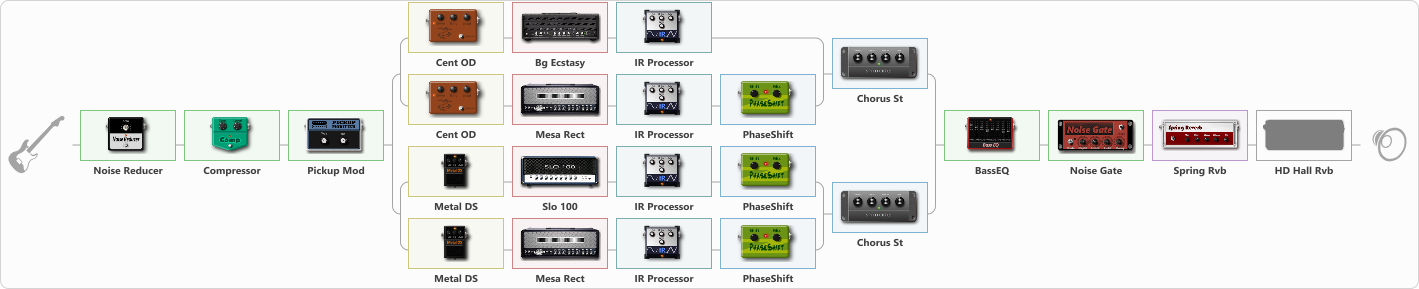# Metal/Djent Practice Setup

Discussion in 'ToneLib-GFX presets' started by Eric Mills, Dec 23, 2020.

1. Metal/Djent Practice Setup

Preset name: Metal/Djent Practice

Tons of gain and distortion. Great for practicing riffs

Effects chain:Effect: "Noise Reducer" (Dynamics / Filter), active - "yes"
{
"Sens" = 80
"Mode" = Soft
}

Effect: "Compressor" (Dynamics / Filter), active - "yes"
{
"Sense" = 38
"Level" = 50
}

Effect: "Pickup Mod" (Dynamics / Filter), active - "yes"
{
"Mode" = Single->Humbacker
"Tone" = 50
}

Effect: "Splitter" (Dynamics / Filter), active - "yes"
{
"A-Bypass" = Off
"A-Pan" = 0
"A-Level" = 55
"B-Bypass" = Off
"B-Pan" = 0
"B-Level" = 55

'A' branch:
{

Effect: "Splitter" (Dynamics / Filter), active - "yes"
{
"A-Bypass" = Off
"A-Pan" = 0
"A-Level" = 55
"B-Bypass" = Off
"B-Pan" = 0
"B-Level" = 55

'A' branch:
{

Effect: "Cent OD" (Overdrive / Distortion), active - "yes"
{
"Drive" = 68
"Tone" = 42
"Level" = 59
}

Effect: "Bg Ecstasy" (Amp simulators), active - "yes"
{
"Gain" = 66
"Bass" = 38
"Middle" = 81
"Treble" = 62
"Presence" = 59
"Master" = 66
"Level (dB)" = 1
}

Effect: "IR Processor" (Cabinets), active - "yes"
{
"IR" = Hesu 2x12 V30 - 440 center edge
"Low Cut (Hz)" = 80
"Hi Cut (kHz)" = 8.0
"Mix" = 100
"Level (dB)" = 1
}
}
'B' branch:
{

Effect: "Cent OD" (Overdrive / Distortion), active - "yes"
{
"Drive" = 68
"Tone" = 42
"Level" = 59
}

Effect: "Mesa Rect" (Amp simulators), active - "yes"
{
"Gain" = 66
"Bass" = 38
"Middle" = 81
"Treble" = 62
"Presence" = 59
"Master" = 66
"Level (dB)" = 1
}

Effect: "IR Processor" (Cabinets), active - "yes"
{
"IR" = ML Sound Lab's BEST IR IN THE WORLD
"Low Cut (Hz)" = 80
"Hi Cut (kHz)" = 12.0
"Mix" = 100
"Level (dB)" = 4
}

Effect: "PhaseShift" (Modulation / Sfx), active - "yes"
{
"Shift" = 36
"Mix" = 100
}
}
}

Effect: "Chorus St" (Modulation / Sfx), active - "yes"
{
"Speed" = 1.9
"Depth" = 31
"Center" = 4.4
"Mix" = 40
}
}
'B' branch:
{

Effect: "Splitter" (Dynamics / Filter), active - "yes"
{
"A-Bypass" = Off
"A-Pan" = 0
"A-Level" = 55
"B-Bypass" = Off
"B-Pan" = 0
"B-Level" = 55

'A' branch:
{

Effect: "Metal DS" (Overdrive / Distortion), active - "yes"
{
"Dist" = 64
"Bass" = 61
"Middle" = 12
"Treble" = 56
"Level" = 46
}

Effect: "Slo 100" (Amp simulators), active - "yes"
{
"Gain" = 66
"Bass" = 38
"Middle" = 81
"Treble" = 62
"Presence" = 59
"Master" = 66
"Level (dB)" = 1
}

Effect: "IR Processor" (Cabinets), active - "yes"
{
"IR" = ML Sound Lab's BEST IR IN THE WORLD
"Low Cut (Hz)" = 0
"Hi Cut (kHz)" = 20.0
"Mix" = 100
"Level (dB)" = 0
}

Effect: "PhaseShift" (Modulation / Sfx), active - "yes"
{
"Shift" = 36
"Mix" = 100
}
}
'B' branch:
{

Effect: "Metal DS" (Overdrive / Distortion), active - "yes"
{
"Dist" = 64
"Bass" = 61
"Middle" = 12
"Treble" = 56
"Level" = 46
}

Effect: "Mesa Rect" (Amp simulators), active - "yes"
{
"Gain" = 66
"Bass" = 38
"Middle" = 81
"Treble" = 62
"Presence" = 59
"Master" = 66
"Level (dB)" = 1
}

Effect: "IR Processor" (Cabinets), active - "yes"
{
"IR" = Hesu 2x12 V30 - 440 center edge
"Low Cut (Hz)" = 0
"Hi Cut (kHz)" = 20.0
"Mix" = 100
"Level (dB)" = 0
}

Effect: "PhaseShift" (Modulation / Sfx), active - "yes"
{
"Shift" = 36
"Mix" = 100
}
}
}

Effect: "Chorus St" (Modulation / Sfx), active - "yes"
{
"Speed" = 1.9
"Depth" = 31
"Center" = 4.4
"Mix" = 40
}
}
}

Effect: "BassEQ" (Dynamics / Filter), active - "yes"
{
"50 Hz" = 10
"120 Hz" = -8
"400 Hz" = -4
"500 Hz" = -2
"800 Hz" = 3
"4.5 kHz" = 8
"10 kHz" = 0
"Level (dB)" = 2
}

Effect: "Noise Gate" (Dynamics / Filter), active - "yes"
{
"Mode" = Auto
"Depth" = 53
"Threshold" = 46
"Attack" = 52
"Hold" = 269
"Decay" = 330
}

Effect: "Spring Rvb" (Reverberation), active - "yes"
{
"Time" = 7.0
"PreDelay" = 0
"LoDamp" = 16
"HiDamp" = 26
"Mix" = 42
}

Effect: "HD Hall Rvb" (Reverberation), active - "no"
{
"Time" = 7.7
"PreLPF" = 44
"PreDelay" = 96
"HiDamp" = 27
"LoGain" = -3.3
"Mix" = 48
}

Note: You will need to download and install the ToneLib-GFX software to use the preset.

File size:
57.1 KB
Views:
2,953